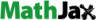## Friday, July 26, 2013

### How to add Mathematical Formulas to a Blog or Webpage

I've recently been working on a mathematical derivation of the Poisson Distribution and am nearing its completion. As I am planning to document this information, I realized that the simplest explanation is to illustrate the underlying formulas.Through my browsing, I found a couple of webpages that include various formulas. By accident, I happened to "right click" on one of them and was presented with a pop-up menu from MathJax.

Following the links, I learned that MathJax is exactly what I need.

For example, the Poisson distribution formula is:

P(X)=(e^-mu mu^x)/(x!)

To get this to display correctly in Blogger or a webpage, two things must first be done.

First: The template must be edited and a CDN link to MathJax must be added. The line to be added is:

<script src="http://cdn.mathjax.org/mathjax/latest/MathJax.js?config=AM_HTMLorMML-full" type="text/javascript">

and it should be placed just before the </head> tag. Once you have added this line, make sure you save the blog template or webpage. This only has to be done once in each blog or webpage where you want to display formulas.

Second: Now that this is complete, you may now enter formulas.  This should always be done in raw HTML mode (but this is not necessary in Blogger).

The syntax for MathJax is very similar to mathematical expressions when using ASCII Math notation. The html code for the above Poisson formula is:
P(X)=(e^-mu mu^x)/(x!)
The formula will remain in this format when you are editing the webpage or blog post. But, when you display it after it is published and viewed in a browser, the MathJax script will translate the HTML syntax into the corresponding  mathematical formula.